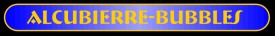Time Travel Research Center © 1998  Cetin BAL - GSM:+90  05366063183 - Turkey / DenizliOne of the oldest transportation facilities are ships. They use wind or current for their movement. A space-ship could do the same provided it were possible to construct a spacetime containing a 'stream' that carries the object in question at a sufficiently high velocity with respect to some point outside the 'stream'. This is achieved by using the metric suggested by Alcubierre. In the coordinates (t, x, y, z) it can be written as follows:For an observer in the middle of this 'stream' (i.e. y = z = 0) it is clear that gamma(t) = (t, vs t) is a time-like path for dvs/dt = 0. Here vs is an arbitrary parameter, which can be interpreted as constant velocity. In particular vs can be bigger than 1, thus exceeding the speed of light (in natural units). For vs = 0 or fs = 0 the Lorentz metric is recovered. The function fs(rs) controls the shape of the 'stream'. It depends on rs only, the distance from the curve x = y = z = 0. For rs > R we want fs = 0, for rs < R - sigma we want fs = 1. So fs may look like this:The spatial part of this metric hence describes a 'bubble' with radius R and walls of the width s. Adopting the denotation from popular science this may be called a warp bubble. As fr is approximately constant in the interior, tidal forces will be very small thus an extended observer such as a space-ship can be placed in the center without danger.Of course this spacetime causes a deviation of light. In the case of a warp bubble moving upwards, light rays coming from the exterior region will be deflected and blue-shifted as viewed from the center. This is shown in the diagrams below (left: vs ~ 2, right: vs ~ 4). Photons coming directly from below are infinitely red-shifted and are hence not observable. So there exists a past event horizon (and analogously a future event horizon in the opposite direction) indicated by the shaded region. The travellers inside the bubble will see a fixed star background like in the middle part of the diagram. From the Einstein equations the energy-momentum-tensor can be calculated. From this the energy density and matter distribution are known. Once calculated, it is obvious that a negative energy density is necessary. The diagrams below show the cases vs ~ 1 (left) and vs ~ 5 (right).Parts of the bubble are causally disconnected from the center. Hence the crew of the space-ship cannot control its way - the route must be planned by an observer in the causal past of the complete path. Therefore the Alcubierre metric rather describes a 'trolley'. Another problem with this metric is the occurence of closed time-like curves. This means that the flow of information is not only future directed. Hence the principle of causality is not fulfilled any more and the topology of the spacetime becomes non trivial. Thus the question is raised: is it principally possible to modify spacetime as indicated? Hiçbir yazý/ resim  izinsiz olarak kullanýlamaz!!  Telif haklarý uyarýnca bu bir suçtur..! Tüm haklarý Çetin BAL' a aittir. Kaynak gösterilmek ţartýyla  siteden alýntý yapýlabilir.  © 1998  Cetin BAL - GSM:+90  05366063183 - Turkiye / Denizli  Ana Sayfa /Index /Roket bilimi / E-Mail / Rölativite Dosyasý     Time Travel Technology /UFO Galerisi /UFO Technology/ Kuantum Teleportation /Kuantum Fizigi /Uçaklar(Aeroplane)